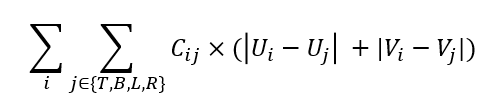# How to customize/define a loss function like this?

I have a network and the input has the shape like: batch x 6 x 180 x 320 ( 6channels ), after several layers of convolutions and convolution transpose, the output is of the shape: batch x 2 x180 x 320(2channels).
Suppose the element of the first channel is U, and the second channel is V,
and I want to define a loss like:i is the i th element of the second channel of the output, j denotes his 4 surrounding neighbor elements(left bot, up, right)
Cij is a weight calculated from the input.
So is there a sufficient way to calculate this loss, I’ve read autograd
But according to my loss definition, I think I have to iterate the elements of two channels but is it possible?
Thanks!

For efficiency, you’ll want to use tensor operations instead of looping over every element.

Something like:

``````output = ... # batch x 2 x 180 x 320
u = output[:, 0, :, :]
v = output[:, 1, :, :]

def delta_neighbors(x):
assert x.dim() == 3
x = x.unsqueeze(1)  # view as 4D: batch x 1 x h x w
return (padded - top).abs() * c + (padded - bottom).abs() * c + (padded - left).abs() * c + (padded - right).abs() * c

loss = delta_neighbors(u).sum() + delta_neighbors(v).sum()
``````

Thanks! I will try it.

I find the size of x is equal to top , bot ,left ,right not padded
so

output = … # batch x 2 x 180 x 320
u = output[:, 0, :, :]
v = output[:, 1, :, :]

def delta_neighbors(x):
assert x.dim() == 3
x = x.unsqueeze(1) # view as 4D: batch x 1 x h x w